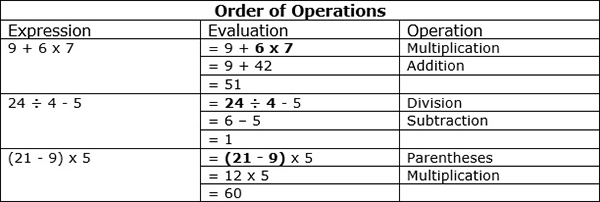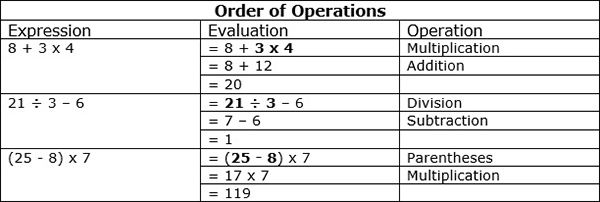# Introduction to Order of Operations

When performing arithmetic operations, a set of rules are used in order to have clarity and avoid confusion. Mathematicians have come up with a standard order of operations for calculations involving more than one arithmetic operation. This order of operations is given by Parentheses, Exponent, Multiplication, Division, Addition and Multiplication (PEMDAS) Rule.

• So the first thing we do is evaluate expressions within parentheses.
• Next we evaluate terms with exponents.
• Then we multiply and divide working from left to right.
• Lastly we add and subtract working from left to right.

If any of the steps do not apply, we skip to next step in the order and proceed further.

Evaluate the following using the order of operations

1. 9 + 6 × 7

2. 24 ÷ 4 – 5

3. (21 - 9) × 5

### SolutionEvaluate the following using the order of operations

1. 8 + 3 × 4

2. 21 ÷ 3 – 6

3. (25 - 8) × 7

### Solution